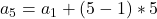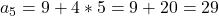## Write the recursive rule for the nth term. Then find the 5th term. {9, 14, 19…]

Question

Write the recursive
rule for the nth term.
Then find the 5th term.
{9, 14, 19…]

in progress 0
7 months 2021-08-02T07:41:16+00:00 1 Answers 3 views 0

Step-by-step explanation:

{9, 14, 19, …} so it looks like 5 is added to the previous term to get next

The recursive rule may be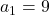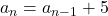The fith term is you find by writing the arithmetic sequence formula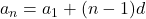, where d is how much you add

yet for the 5th term